|

# 连厕所都没有的上海老别墅,1月爆改后终于甩掉夜壶,用上卫生间,走向人生巅峰!

房君已经好久没有分享过《生活改造家》的案例了，但是这次要分享的案例，房君真的觉得很佩服。

背景

▽

这是一个二战时期犹太人的避难所（大别墅），一层是会客厅，二层是主人房，三层是佣人房，后被改成市民住房。

这个五口之家的公婆、夫妻和婴儿，住在第三层中，这层一共住了五户人家十五口人，房主的家在西南（左下角）厕，公共厕所和公共厨房在西北侧。

改造前的

公共区域

▽

主持人和设计师走进这个年久的洋房，微微颤抖的木质楼梯狭窄且陡，两边墙壁斑驳墙皮脱落，因为脏乱差鼠患问题严重，走过这一条长长的楼梯走廊，就像走过了半个世纪前的上海。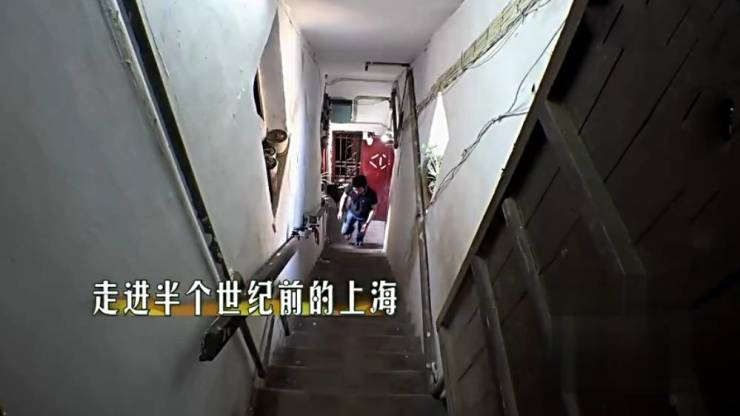在上楼梯的时候，主持人和设计师就被辣椒的味道呛得咳嗽，弥漫的辣味和烟味提醒了他们，这个地方的厨房通风极差，果然走进去他们看到了一个局促、油腻且破旧的厨房。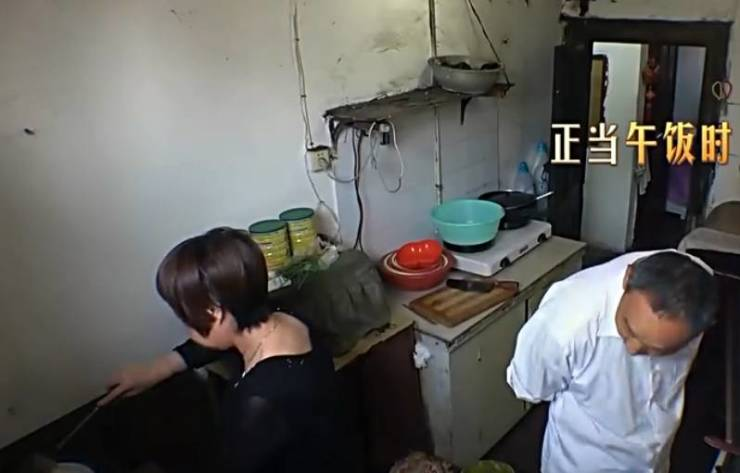厨房里有四个灶台，四个邻居共用，做饭的时候转个身都会碰到别人，狭小的空间造成了诸多安全隐患。

没地方放菜板、拿刀说话的时候可能会碰到别人、裸露的插座开关油腻堆积，没有水槽洗菜要去旁边的卫生间，厨房的窗户不开油烟无处安放，开了又会吹灭煤气，每次做饭就像一场肉搏。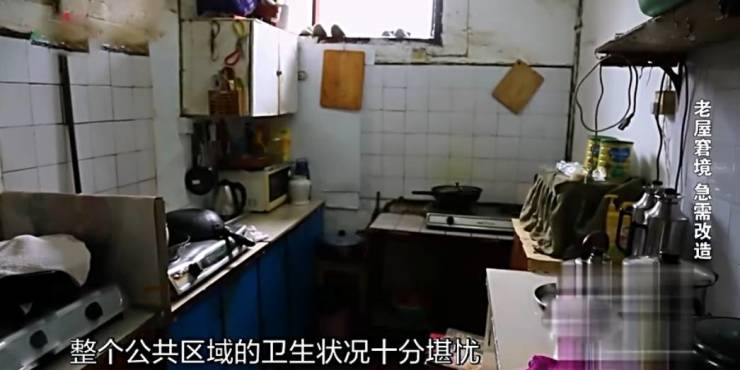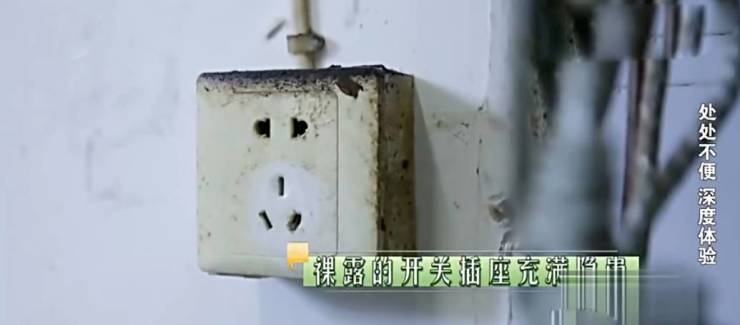卫生间只有一个帘子作为遮挡，主持人在掀开帘子时有一个老头在里面洗澡，场面一度十分尴尬。洗菜也要在卫生间，设计师在体验过程中，洗菜的时候遇到邻居来倒用了一夜的痰盂，他觉得洗菜的时候倒痰盂很不卫生，但又没办法，因为只有一个排水口。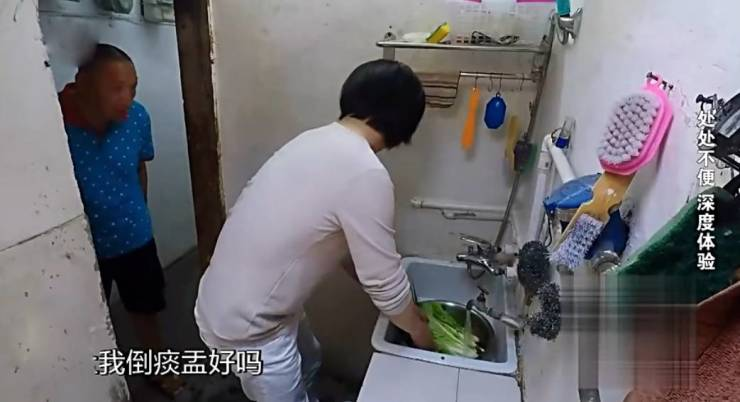多年来这些人就过着一边有人在洗菜，一边有人在另一边洗痰盂洗马桶的生活，在洗菜盆边冲马桶这个点让设计师内心有点崩溃。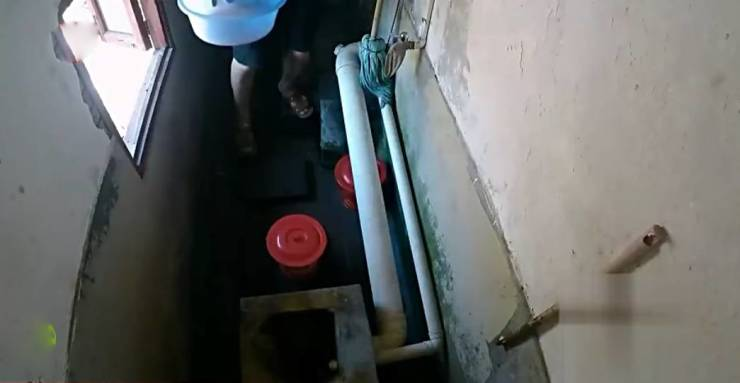砖木结构的老房，没有科学的上下水和排风系统，公共区域的卫生状况和安全隐患堪忧。

改造前的

房主房间

▽

客厅是整个一家人的公共区域，只有6㎡，层高1.8米，承载着客厅、餐厅、和婴儿房的功能，拥挤的房间里堆满了婴儿用品，使用起来只能像“华容道”一样挪出位置。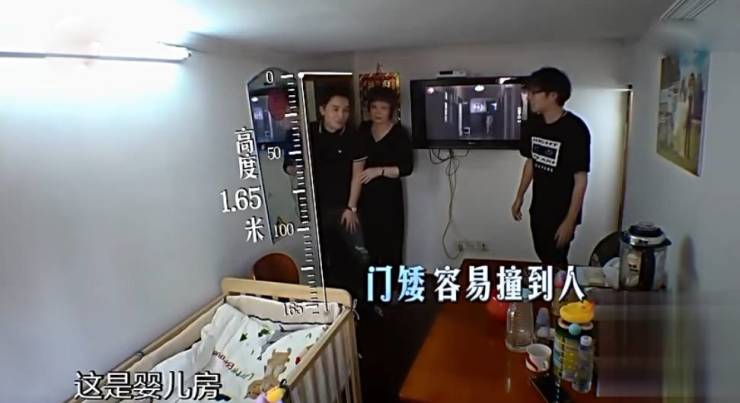吃饭的时候只有四把椅子，来客人的时候就出去吃。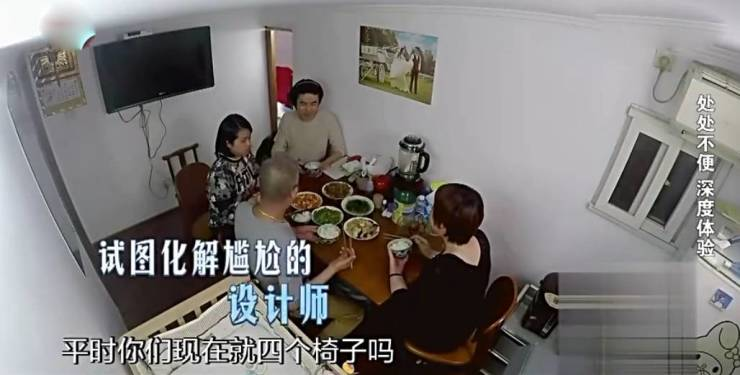客厅里的一个小房间，是儿子和儿媳的卧室。

房间很低，面积约6㎡，层高最矮处只有1.55米，是设计组至今遇到的最矮的房型，在房间里要一直弯着腰，房门也很窄，出入需要侧身。

天花板是楼上邻居的一个窗户，抬高的话会影响隔壁，因为是老房子，不能抬高也不能深挖。

低矮的房间使得空调的出风口只能正对着人脸，因为房间的挑高低面积小，整个家庭的氛围就很压抑。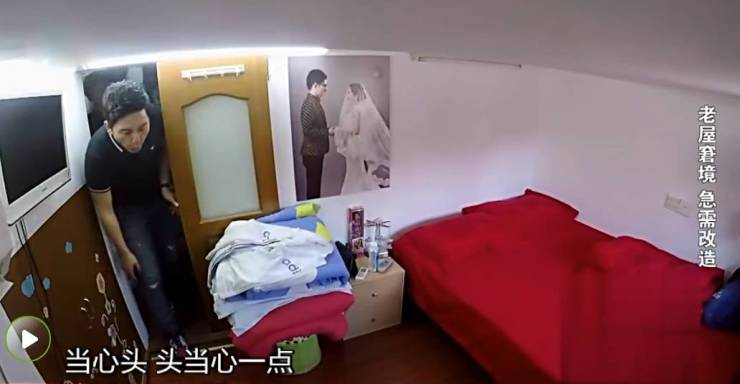不足8㎡，摆下床和柜子后人活动的面积只有1㎡左右。

但是设计师仿佛有魔力般，经过一个月的改造，在不能改变房屋结构的基础上，给这个老房子里，打造出独立的卫生间、婆婆洗衣服的区域和独立的客厅。

同时，公共区域的厨房和卫生间也被设计师改造了。

改造后的

公共区域

▽

卫生间装了门，单独设置了一个淋浴区，以浴帘相隔，解决了洗澡时有人误入的窘迫。功能分区被重做，外部是洗漱区，内部是淋浴区，因为每家的生活习惯不同，排污口就只做了基本改造，视觉上看起来干净利索，新增的自动消毒清新装置，让公共洗手间更加卫生。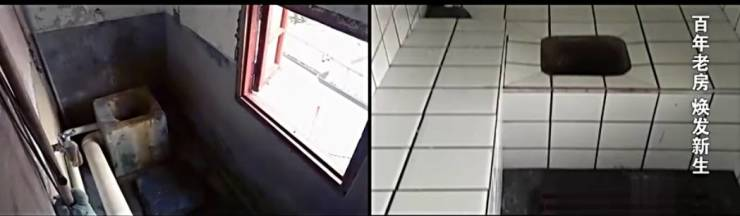房间也装了一扇门，重新装了油烟机，一家一户自己用自己的电。

石英石台面容易打理，地砖特意挑选了防滑防油腻的。

厨房里还加了利用超声波干扰老鼠的捕鼠器，解决了鼠患问题。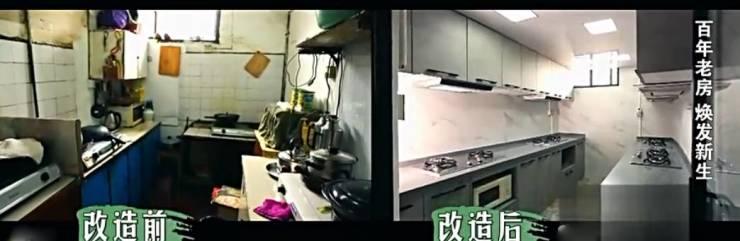煤气灶选择了不容易被吹灭的， 为了以防万一增加了燃气漏气报警装置。

原本厨房一家独大的布局让其他人家连案板都难以搁置，改造后每家的面积基本一致，不但操作起来不会彼此干扰，还多出了放置微波炉的空间。蓝色的复古地板，为了搭配老洋房原本的风格，楼梯彩绘、增加了灯带提亮了楼梯间的视觉效果，同时墙面也做了花鸟的装饰。

为了增加楼梯的宽度，设计师改变了楼梯的坡度，这也是室内楼梯最舒适的坡度。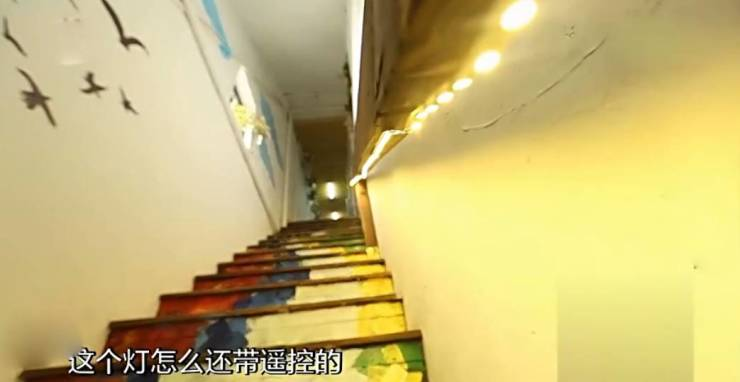改造后的

房主房间

▽

进门处的公共空间是客厅，兼具了吃饭和会客的功能，电视在沙发对面，平时还可以坐在这里看看电视节目。

阳台被做成了洗衣区，洗衣机和水槽被放在里面，婆婆洗衣服的时候再也不用从走廊挪动洗衣机到卫生间了。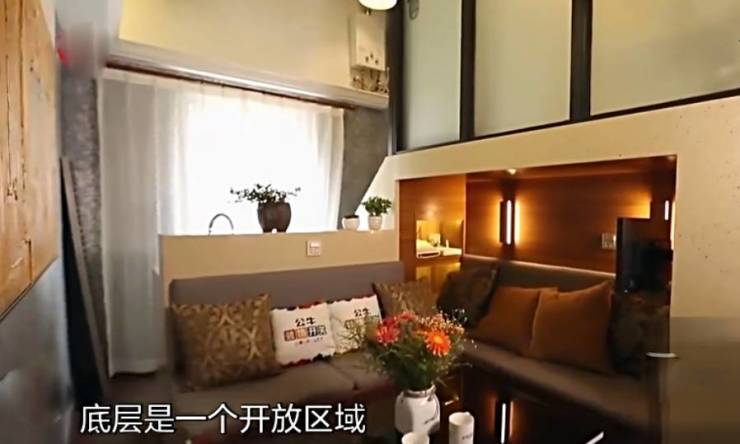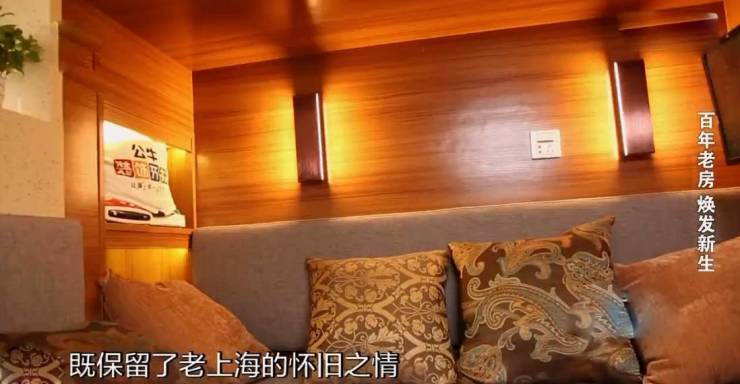客厅是原本的主卧，被拆除变成一个小loft，底层是一个开放式区域成为全家人吃饭会客的场所，上层是卫生间。原本需要挣扎着入座的餐桌不见了，取而代之的是温馨宽松的就餐环境，卡座背面简易操作台和水槽，在这里制作婴儿辅食十分卫生便捷。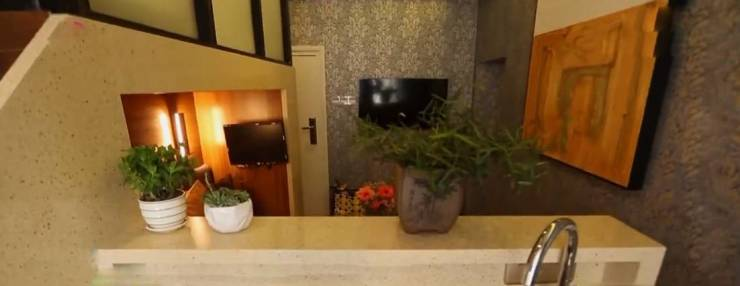客厅的茶几用遥控器控制自动升降，会客是茶几的高度，吃饭是餐桌的高度，中间是个电磁炉，可以直接吃火锅。冰箱隐藏在柜子中，外面看起来像个储物柜，其实是和柜子结合在一起，关门的时候就是个柜子，容积也变大，从后面散热，不用担心冰箱散热的问题。被改造成榻榻米的样子，使房间的层高在视觉上看起来更高，空间感大大提高，又因为优化了收纳方案，空间显得更加通透宽敞。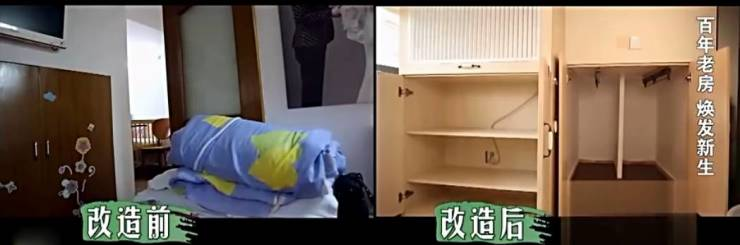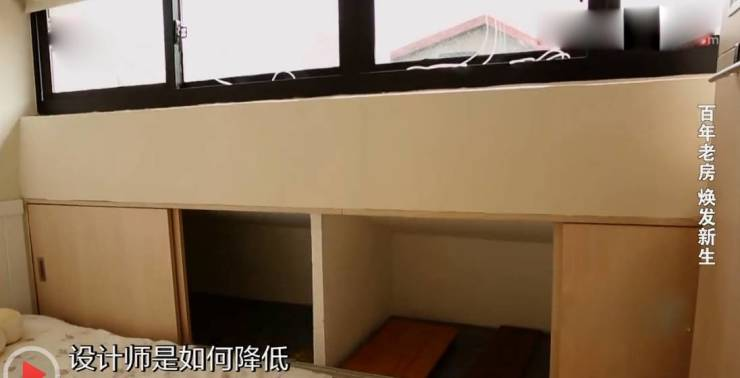在层高不能改变的前提下，设计师降低了空间压抑感。既然不能改变高度，就进屋直接坐下。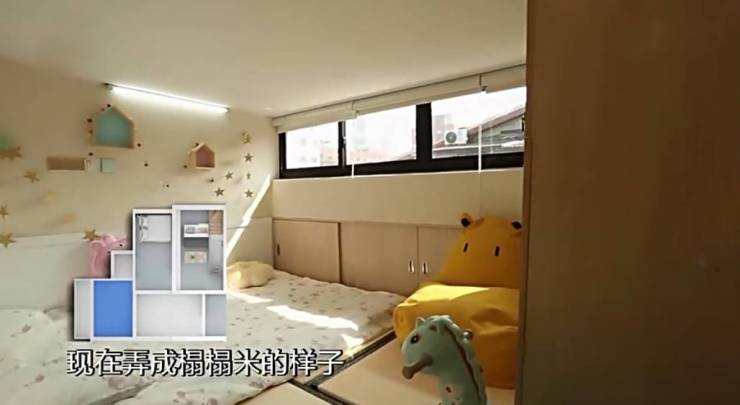曾经阁楼下面的空间变成了储藏室，可以挂衣服，一米二的深度，设计了伸缩式挂衣杆，可以拉长，方便使用。卫生间就设置在客厅的二层，一家人可以享受独立的卫生间，还能在里面洗澡，这大概是十几年来这家人几乎没有享受过的了。经过严格的防水和避电处理，这里也是宝宝洗澡的地方。

电子马桶可以打碎废纸，通过水管排出去。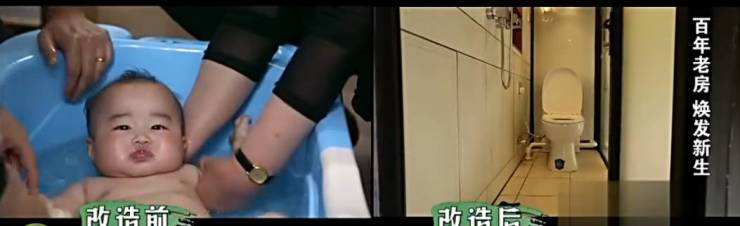原本的床被被换成一张大床，阳光透过窗户的时候，屋子也是宽敞明亮。`声明：本文由入驻焦点开放平台的作者撰写，除焦点官方账号外，观点仅代表作者本人，不代表焦点立场错误信息举报电话： 400-099-0099，邮箱：jubao@vip.sohu.com，或点此进行意见反馈，或点此进行举报投诉。`A B C D E F G H J K L M N P Q R S T W X Y Z
A - B - C - D - E
• A
• 鞍山
• 安庆
• 安阳
• 安顺
• 安康
• 澳门
• B
• 北京
• 保定
• 包头
• 巴彦淖尔
• 本溪
• 蚌埠
• 亳州
• 滨州
• 北海
• 百色
• 巴中
• 毕节
• 保山
• 宝鸡
• 白银
• 巴州
• C
• 承德
• 沧州
• 长治
• 赤峰
• 朝阳
• 长春
• 常州
• 滁州
• 池州
• 长沙
• 常德
• 郴州
• 潮州
• 崇左
• 重庆
• 成都
• 楚雄
• 昌都
• 慈溪
• 常熟
• D
• 大同
• 大连
• 丹东
• 大庆
• 东营
• 德州
• 东莞
• 德阳
• 达州
• 大理
• 德宏
• 定西
• 儋州
• 东平
• E
• 鄂尔多斯
• 鄂州
• 恩施
F - G - H - I - J
• F
• 抚顺
• 阜新
• 阜阳
• 福州
• 抚州
• 佛山
• 防城港
• G
• 赣州
• 广州
• 桂林
• 贵港
• 广元
• 广安
• 贵阳
• 固原
• H
• 邯郸
• 衡水
• 呼和浩特
• 呼伦贝尔
• 葫芦岛
• 哈尔滨
• 黑河
• 淮安
• 杭州
• 湖州
• 合肥
• 淮南
• 淮北
• 黄山
• 菏泽
• 鹤壁
• 黄石
• 黄冈
• 衡阳
• 怀化
• 惠州
• 河源
• 贺州
• 河池
• 海口
• 红河
• 汉中
• 海东
• 怀来
• I
• J
• 晋中
• 锦州
• 吉林
• 鸡西
• 佳木斯
• 嘉兴
• 金华
• 景德镇
• 九江
• 吉安
• 济南
• 济宁
• 焦作
• 荆门
• 荆州
• 江门
• 揭阳
• 金昌
• 酒泉
• 嘉峪关
K - L - M - N - P
• K
• 开封
• 昆明
• 昆山
• L
• 廊坊
• 临汾
• 辽阳
• 连云港
• 丽水
• 六安
• 龙岩
• 莱芜
• 临沂
• 聊城
• 洛阳
• 漯河
• 娄底
• 柳州
• 来宾
• 泸州
• 乐山
• 六盘水
• 丽江
• 临沧
• 拉萨
• 林芝
• 兰州
• 陇南
• M
• 牡丹江
• 马鞍山
• 茂名
• 梅州
• 绵阳
• 眉山
• N
• 南京
• 南通
• 宁波
• 南平
• 宁德
• 南昌
• 南阳
• 南宁
• 内江
• 南充
• P
• 盘锦
• 莆田
• 平顶山
• 濮阳
• 攀枝花
• 普洱
• 平凉
Q - R - S - T - W
• Q
• 秦皇岛
• 齐齐哈尔
• 衢州
• 泉州
• 青岛
• 清远
• 钦州
• 黔南
• 曲靖
• 庆阳
• R
• 日照
• 日喀则
• S
• 石家庄
• 沈阳
• 双鸭山
• 绥化
• 上海
• 苏州
• 宿迁
• 绍兴
• 宿州
• 三明
• 上饶
• 三门峡
• 商丘
• 十堰
• 随州
• 邵阳
• 韶关
• 深圳
• 汕头
• 汕尾
• 三亚
• 三沙
• 遂宁
• 山南
• 商洛
• 石嘴山
• T
• 天津
• 唐山
• 太原
• 通辽
• 铁岭
• 泰州
• 台州
• 铜陵
• 泰安
• 铜仁
• 铜川
• 天水
• 天门
• W
• 乌海
• 乌兰察布
• 无锡
• 温州
• 芜湖
• 潍坊
• 威海
• 武汉
• 梧州
• 渭南
• 武威
• 吴忠
• 乌鲁木齐
X - Y - Z
• X
• 邢台
• 徐州
• 宣城
• 厦门
• 新乡
• 许昌
• 信阳
• 襄阳
• 孝感
• 咸宁
• 湘潭
• 湘西
• 西双版纳
• 西安
• 咸阳
• 西宁
• 仙桃
• 西昌
• Y
• 运城
• 营口
• 盐城
• 扬州
• 鹰潭
• 宜春
• 烟台
• 宜昌
• 岳阳
• 益阳
• 永州
• 阳江
• 云浮
• 玉林
• 宜宾
• 雅安
• 玉溪
• 延安
• 榆林
• 银川
• Z
• 张家口
• 镇江
• 舟山
• 漳州
• 淄博
• 枣庄
• 郑州
• 周口
• 驻马店
• 株洲
• 张家界
• 珠海
• 湛江
• 肇庆
• 中山
• 自贡
• 资阳
• 遵义
• 昭通
• 张掖
• 中卫

1室1厅1厨1卫1阳台

1
2
3
4
5

0
1
2

1

1

0
1
2
3报名成功，资料已提交审核A B C D E F G H J K L M N P Q R S T W X Y Z
A - B - C - D - E
• A
• 鞍山
• 安庆
• 安阳
• 安顺
• 安康
• 澳门
• B
• 北京
• 保定
• 包头
• 巴彦淖尔
• 本溪
• 蚌埠
• 亳州
• 滨州
• 北海
• 百色
• 巴中
• 毕节
• 保山
• 宝鸡
• 白银
• 巴州
• C
• 承德
• 沧州
• 长治
• 赤峰
• 朝阳
• 长春
• 常州
• 滁州
• 池州
• 长沙
• 常德
• 郴州
• 潮州
• 崇左
• 重庆
• 成都
• 楚雄
• 昌都
• 慈溪
• 常熟
• D
• 大同
• 大连
• 丹东
• 大庆
• 东营
• 德州
• 东莞
• 德阳
• 达州
• 大理
• 德宏
• 定西
• 儋州
• 东平
• E
• 鄂尔多斯
• 鄂州
• 恩施
F - G - H - I - J
• F
• 抚顺
• 阜新
• 阜阳
• 福州
• 抚州
• 佛山
• 防城港
• G
• 赣州
• 广州
• 桂林
• 贵港
• 广元
• 广安
• 贵阳
• 固原
• H
• 邯郸
• 衡水
• 呼和浩特
• 呼伦贝尔
• 葫芦岛
• 哈尔滨
• 黑河
• 淮安
• 杭州
• 湖州
• 合肥
• 淮南
• 淮北
• 黄山
• 菏泽
• 鹤壁
• 黄石
• 黄冈
• 衡阳
• 怀化
• 惠州
• 河源
• 贺州
• 河池
• 海口
• 红河
• 汉中
• 海东
• 怀来
• I
• J
• 晋中
• 锦州
• 吉林
• 鸡西
• 佳木斯
• 嘉兴
• 金华
• 景德镇
• 九江
• 吉安
• 济南
• 济宁
• 焦作
• 荆门
• 荆州
• 江门
• 揭阳
• 金昌
• 酒泉
• 嘉峪关
K - L - M - N - P
• K
• 开封
• 昆明
• 昆山
• L
• 廊坊
• 临汾
• 辽阳
• 连云港
• 丽水
• 六安
• 龙岩
• 莱芜
• 临沂
• 聊城
• 洛阳
• 漯河
• 娄底
• 柳州
• 来宾
• 泸州
• 乐山
• 六盘水
• 丽江
• 临沧
• 拉萨
• 林芝
• 兰州
• 陇南
• M
• 牡丹江
• 马鞍山
• 茂名
• 梅州
• 绵阳
• 眉山
• N
• 南京
• 南通
• 宁波
• 南平
• 宁德
• 南昌
• 南阳
• 南宁
• 内江
• 南充
• P
• 盘锦
• 莆田
• 平顶山
• 濮阳
• 攀枝花
• 普洱
• 平凉
Q - R - S - T - W
• Q
• 秦皇岛
• 齐齐哈尔
• 衢州
• 泉州
• 青岛
• 清远
• 钦州
• 黔南
• 曲靖
• 庆阳
• R
• 日照
• 日喀则
• S
• 石家庄
• 沈阳
• 双鸭山
• 绥化
• 上海
• 苏州
• 宿迁
• 绍兴
• 宿州
• 三明
• 上饶
• 三门峡
• 商丘
• 十堰
• 随州
• 邵阳
• 韶关
• 深圳
• 汕头
• 汕尾
• 三亚
• 三沙
• 遂宁
• 山南
• 商洛
• 石嘴山
• T
• 天津
• 唐山
• 太原
• 通辽
• 铁岭
• 泰州
• 台州
• 铜陵
• 泰安
• 铜仁
• 铜川
• 天水
• 天门
• W
• 乌海
• 乌兰察布
• 无锡
• 温州
• 芜湖
• 潍坊
• 威海
• 武汉
• 梧州
• 渭南
• 武威
• 吴忠
• 乌鲁木齐
X - Y - Z
• X
• 邢台
• 徐州
• 宣城
• 厦门
• 新乡
• 许昌
• 信阳
• 襄阳
• 孝感
• 咸宁
• 湘潭
• 湘西
• 西双版纳
• 西安
• 咸阳
• 西宁
• 仙桃
• 西昌
• Y
• 运城
• 营口
• 盐城
• 扬州
• 鹰潭
• 宜春
• 烟台
• 宜昌
• 岳阳
• 益阳
• 永州
• 阳江
• 云浮
• 玉林
• 宜宾
• 雅安
• 玉溪
• 延安
• 榆林
• 银川
• Z
• 张家口
• 镇江
• 舟山
• 漳州
• 淄博
• 枣庄
• 郑州
• 周口
• 驻马店
• 株洲
• 张家界
• 珠海
• 湛江
• 肇庆
• 中山
• 自贡
• 资阳
• 遵义
• 昭通
• 张掖
• 中卫• 手机• 分享
• 设计
免费设计
• 计算器
装修计算器
• 入驻
合作入驻
• 联系
联系我们
• 置顶
返回顶部